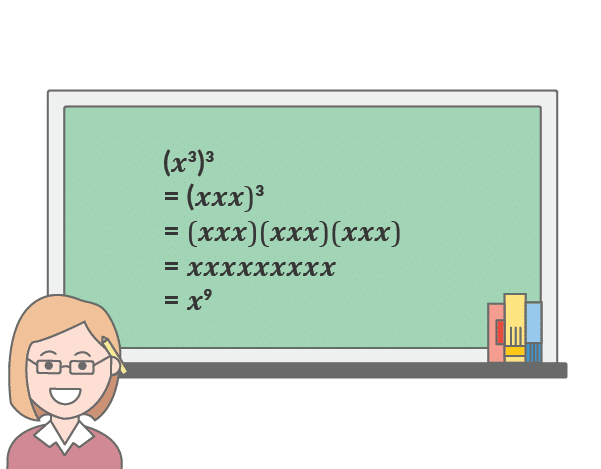# Exponents and their utilization in real life

There are times when multiplying a number represents repeated addition. For example, we can write 2 + 2 + 2 + 2 + 2 = 10. We can simply put this equation as 2 multiplied by 5 which gives the same result. Similarly, an alternative representation can also be used in the case of repeated multiplication.

For example, we can write 2 x 2 x 2 x 2 x 2 = 32. If we try writing more of the twos, then the equation may get too tedious. Thus we use an exponent or a power which helps to multiply the base by the number of times the exponent is denoted. Exponents are generally denoted by a superscript written above the base number. In this article, we would speak about the laws of exponents and their importance.

## What are the laws of exponents?

There are a few laws that are used in finding out the result using exponents. These are also known as properties which we would need to solve using exponents. The following are listed below.

1. Properties of product: xa * xb =xa+b
2. Properties of quotient: xa/xb = xa-b
3. Properties of zero: x0 = 1
4. Properties of negative exponent: x-3=1/x3
5. Properties of power of a power: (xa)b = xab
6. Properties of power of a product: (x * y)a = xaya
7. Properties of power of a quotient: (x/y)a = xa/ya

These are the following properties that are used in determining the results using exponents.

## Objectives of utilizing exponents

• Easily using formulas for repeated multiplication or division for exponential representations.
• Quite simple and easy to find the solution
• Properly evaluate multiple representations and operations using a single expression.

### Utilization in scientific experiments

There are times within science research or a laboratory where you would find scales like the pH meter or Richter scale. Here we can find exponents. Both the apparatuses use logarithms to generally represent a number to its tenth fold place. Chemists and Druggists usually are seen finding out a pH that says 6. It means that the pH of the substance is 106. Similarly, a pH scale of 7 means the pH is about 107.

Geologists similarly use logarithm scales for finding out the seismic activity using a Richter scale. A value of 5 on the Richter scale represents 105 of seismic energy whereas a value of 6 on the same represents 106 of seismic energy. Thus the second earthquake is ten times stronger than the first earthquake.

### Composing smaller or larger numbers

Individuals in the science field, sometimes use exponents to write down a larger number into a smaller one. For example, 19567 can be written as 1.9567 x 104 in scientific causes. Similarly, a smaller number can also be represented using exponents. For example, .082 can be written as 82 x 10-3.

## Calculating measurements

The most common example of finding exponents in real life is to take measurements or finding out a multi-dimensional area. The area is generally measured by using the length and breadth of an element. Thus the unit utilized is known as square feet or square meters. For example, if we measure a house or an apartment, it gives the value in square feet or ft2 which uses an exponent.

Similarly, when we need to find out a volume, we have a three-dimensional view of it which represents length, breadth, and height. So, the unit that we use in representing a volume is denoted by cubic feet or cubic meters. For example, if we need to find the volume of a cylinder, we use the unit cubic feet or ft3 which also uses an exponent.

These are the basics of Exponents. To find out more, do visit Cuemath, one of the best online platforms for math and coding.